# NCERT Solutions for Class 10 Maths Exercise 15.2## myCBSEguide App

CBSE, NCERT, JEE Main, NEET-UG, NDA, Exam Papers, Question Bank, NCERT Solutions, Exemplars, Revision Notes, Free Videos, MCQ Tests & more.

NCERT solutions for Maths Probability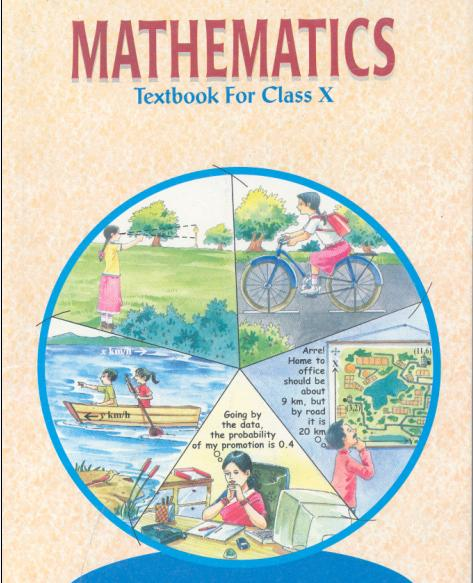## NCERT Solutions for Class 10 Maths Probability

###### 1. Two customers Shyam and Ekta are visiting a particular shop in the same week (Tuesday to Saturday). Each is equally likely to visit the shop on any day as on another day. What is the probability that both will visit the shop on (i) the same day? (ii) consecutive days? (iii) different days?

Ans. Total favourable outcomes associated to the random experiment of visiting a particular shop in the same week (Tuesday to Saturday) by two customers Shyam and Exta are:

(T, T) (T, W) (T, TH) (T, F) (T, S)

(W, T) (W, W) (W, TH) (W, F) (W, S)

(TH, T) (TH, W) (TH, TH) (TH, F) (TH, S)

(F, T) (F, W) (F, TH) (F, F) (F, S)

(S, T) (S, W) (S, TH) (S, F) (S< S)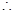Total number of favourable outcomes = 25

(i) The favourable outcomes of visiting on the same day are (T, T), (W, W), (TH, TH), (F, F) and (S, S).Number of favourable outcomes = 5

Hence required probability =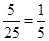(ii) The favourable outcomes of visiting on consecutive days are (T, W), (W, T), (W, TH), (TH, W), (TH, F), (F, TH), (S, F) and (F, S).Number of favourable outcomes = 8

Hence required probability =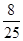(iii) Number of favourable outcomes of visiting on different days are 25 – 5 = 20Number of favourable outcomes = 20

Hence required probability =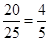NCERT Solutions for Class 10 Maths Exercise 15.2

###### 2. A die is numbered in such a way that its faces show the numbers 1, 2, 2, 3, 3, 6. It is thrown two times and the total score in two throws is noted. Complete the following table which gives a few values of the total score on the two throws: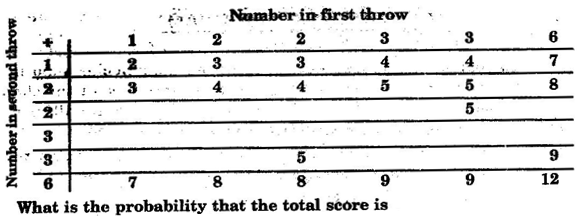What is the probability that the total score is:

(i) even

(ii) 6

(iii) at least 6?

Ans. Complete table is as under: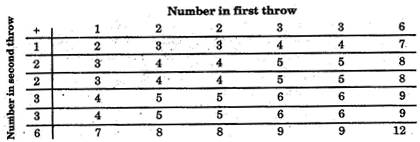It is clear that total number of favourable outcomes =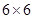= 36

(i) Number of favourable outcomes of getting total score even are 18

Hence P (getting total score even) =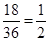(ii) Number of favourable outcomes of getting total score 6 are 4

Hence P (getting total score 6) =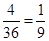(iii) Number of favourable outcomes of getting total score at least 6 are 15

Hence P (getting total score at least 6) =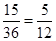NCERT Solutions for Class 10 Maths Exercise 15.2

###### 3. A bag contains 5 red balls and some blue balls. If the probability of drawing a blue ball is double that of a red ball, determine the number of blue balls in the bag.

Ans. Let there be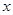blue balls in the bag.Total number of balls in the bag =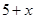Now,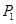= Probability of drawing a blue ball =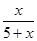And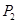= Probability of drawing a blue ball =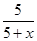But according to question,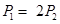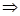=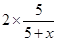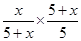=2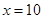Hence, there are 10 blue balls in the bag.

NCERT Solutions for Class 10 Maths Exercise 15.2

###### 4. A box contains 12 balls out of whichare black. If one ball is drawn at random from the box, what is the probability that it will be a black ball?

If 6 more black balls are put in the box, the probability of drawing a black ball is now double of what it was before. Find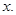Ans. There are 12 balls in the box.

Therefore, total number of favourbale outcomes = 12

The number of favourable outcomes =Therefore= P (getting a black ball) =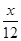If 6 more balls put in the box, then

Total number of favourable outcomes = 12 + 6 = 18

And Number of favourable outcomes =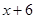P2 = P (getting a black ball) =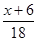According to question,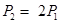=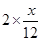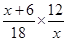=2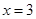NCERT Solutions for Class 10 Maths Exercise 15.2

###### 5. A jar contains 24 marbles, some are green and others are blue. If a marble is drawn at random from the jar, the probability that it is green is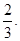Find the number if blue balls in the jar.

Ans. Here, Total number of favourable outcomes = 24

Let there begreen marbles.

Therefore, Favourable number of outcomes =P(G) =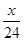But P(G) =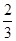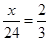= 16

Therefore, number of green marbles are 16

And number of blue marbles = 24 – 16 = 8

## NCERT Solutions for Class 10 Maths Exercise 15.2

NCERT Solutions Class 10 Maths PDF (Download) Free from myCBSEguide app and myCBSEguide website. Ncert solution class 10 Maths includes text book solutions from Mathematics Book. NCERT Solutions for CBSE Class 10 Maths have total 15 chapters. 10 Maths NCERT Solutions in PDF for free Download on our website. Ncert Maths class 10 solutions PDF and Maths ncert class 10 PDF solutions with latest modifications and as per the latest CBSE syllabus are only available in myCBSEguide.

## CBSE app for Class 10

To download NCERT Solutions for Class 10 Maths, Computer Science, Home Science,Hindi ,English, Social Science do check myCBSEguide app or website. myCBSEguide provides sample papers with solution, test papers for chapter-wise practice, NCERT solutions, NCERT Exemplar solutions, quick revision notes for ready reference, CBSE guess papers and CBSE important question papers. Sample Paper all are made available through the best app for CBSE### 3 thoughts on “NCERT Solutions for Class 10 Maths Exercise 15.2”

1. Nice apps the answer is very easy and try to understand easily
THANK YOU

2. Nice apps the answer is very easy and try to understand easily
THANK YOU

3. Thanks aa lot it is the best website to do optional exercsies….